# Unitary matrix

A square matrix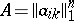over the fieldof complex numbers, whose rows form an orthonormal system, i.e.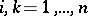. In a unitary space, transformation from one orthonormal basis to another is accomplished by a unitary matrix. The matrix of a unitary transformation relative to an orthonormal basis is also (called) a unitary matrix. A square matrixwith complex entries is unitary if and only if it satisfies any of the following conditions:

1)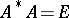;

2);

3)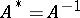;

4) the columns ofform an orthonormal system (here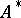is the conjugate transposed of).

The determinant of a unitary matrix is a complex number of modulus one.